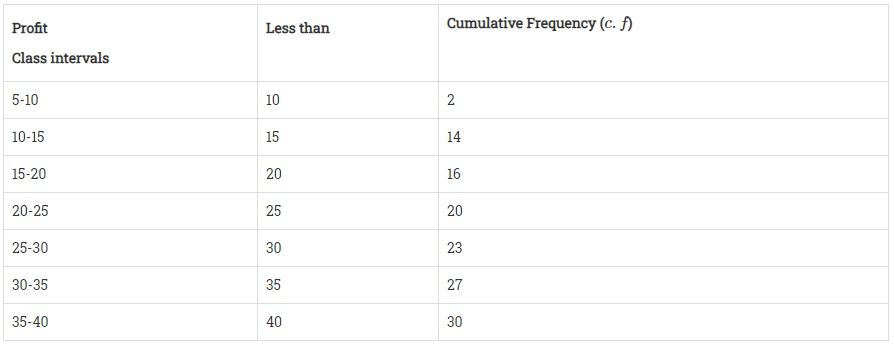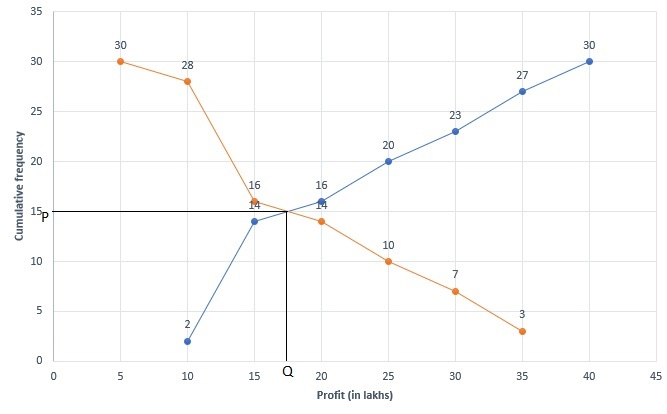# The annual profits earned by 30 shops of a shopping complex in a locality give rise to the following distribution:Profit (in lakhs in Rs)Number of shops (frequency)More than or equal to 530More than or equal to 1028More than or equal to 1516More than or equal to 2014More than or equal to 2510More than or equal to 307More than or equal to 353Draw both ogives for the above data and hence obtain the median.

Given:

The annual profits earned by 30 shops of a shopping complex in a locality give rise
to the given distribution.
To do:

We have to draw both ogives for the above data and hence obtain the median.

Solution:

We first prepare the cumulative  frequency distribution table by more than method as given below:

 Profit (in lakhs in Rs) Number of shops (Cumulative frequency) More than or equal to 5 30 More than or equal to 10 28 More than or equal to 15 16 More than or equal to 20 14 More than or equal to 25 10 More than or equal to 30 7 More than or equal to 35 3

Represent profits(in lakhs) along X-axis and cumulative frequency along Y-axis.

Plot the points (5, 30), (10, 28), (15, 16), (20, 14), (25, 10), (30, 7) and (35, 3) on the graph and join them in free hand to get a more than ogive.

We first prepare the cumulative  frequency distribution table by less than method as given below:Represent profits(in lakhs) along X-axis and cumulative frequency along Y-axis.

Plot the points (10, 3), (15, 7), (20, 10), (25, 14), (30, 16), (35, 28) and (40, 30) on the graph and join them in a free hand to get a less than ogive.Total number of days $N = 30$

This implies,

$\frac{N}{2} = \frac{30}{2}=15$

Draw a line parallel to X-axis at the point of intersection of both ogives, which further intersect at (0, 12) on Y-axis.

Draw a line perpendicular to X-axis at point of intersection of both ogives, which further intersect at (17.50, 0) on X-axis.

Therefore, 17.50 is the required median using ogives.

Hence, the median is 17.50 lakhs.

Updated on: 10-Oct-2022

43 Views